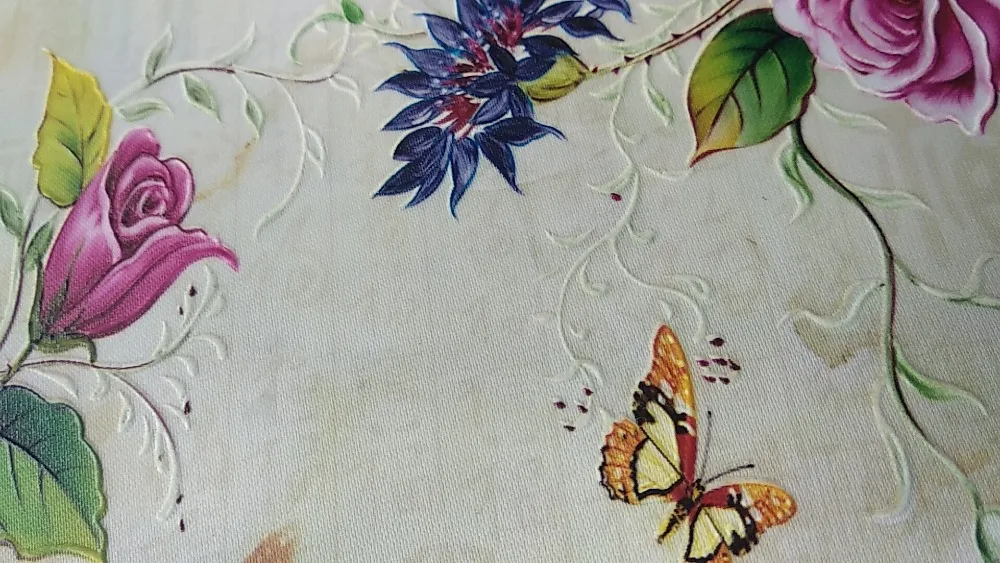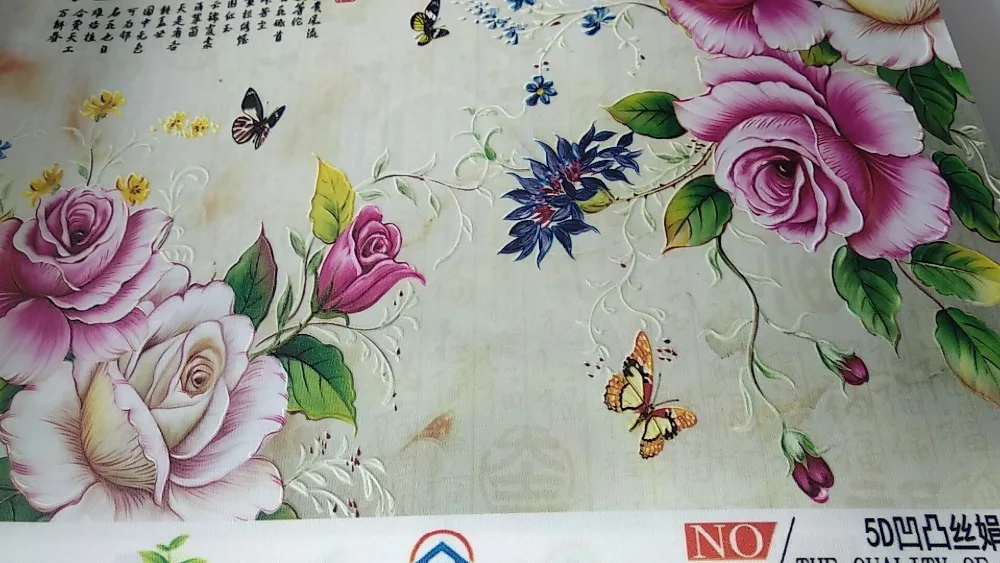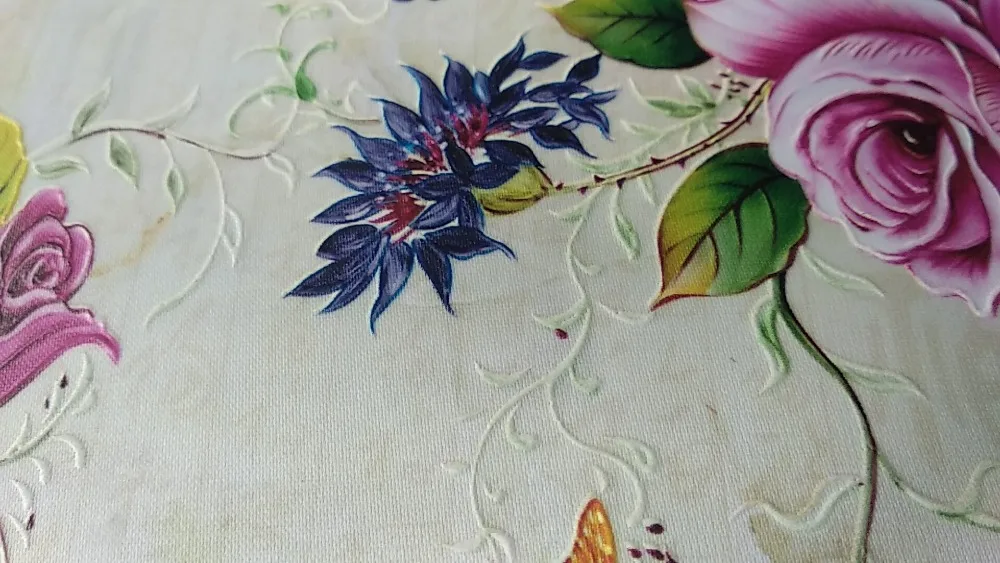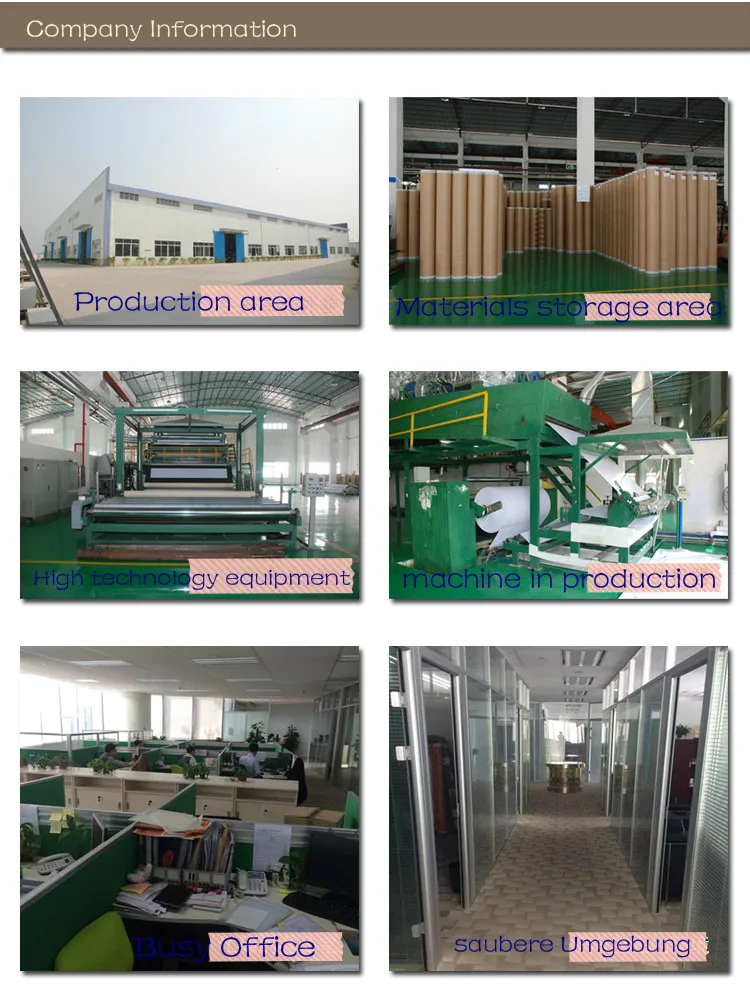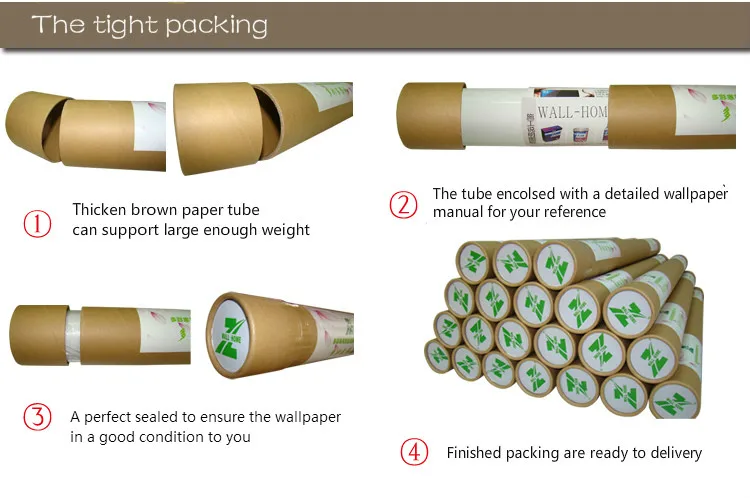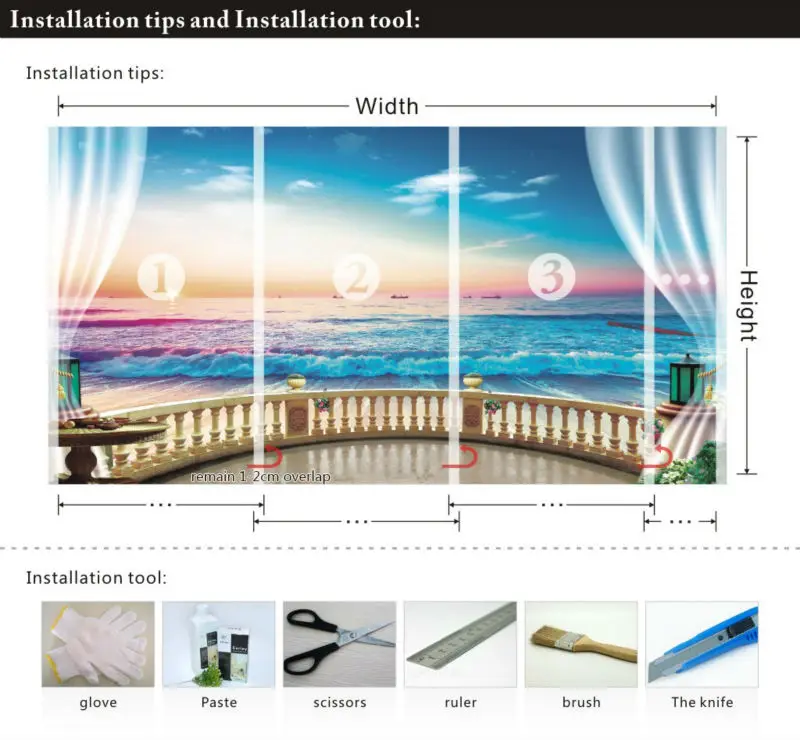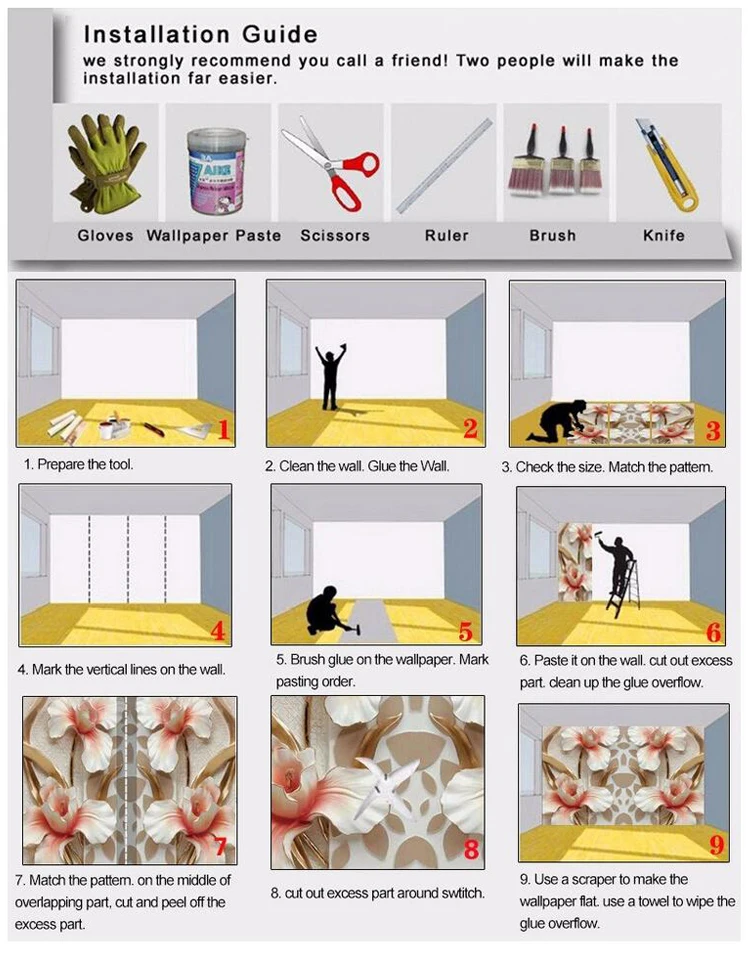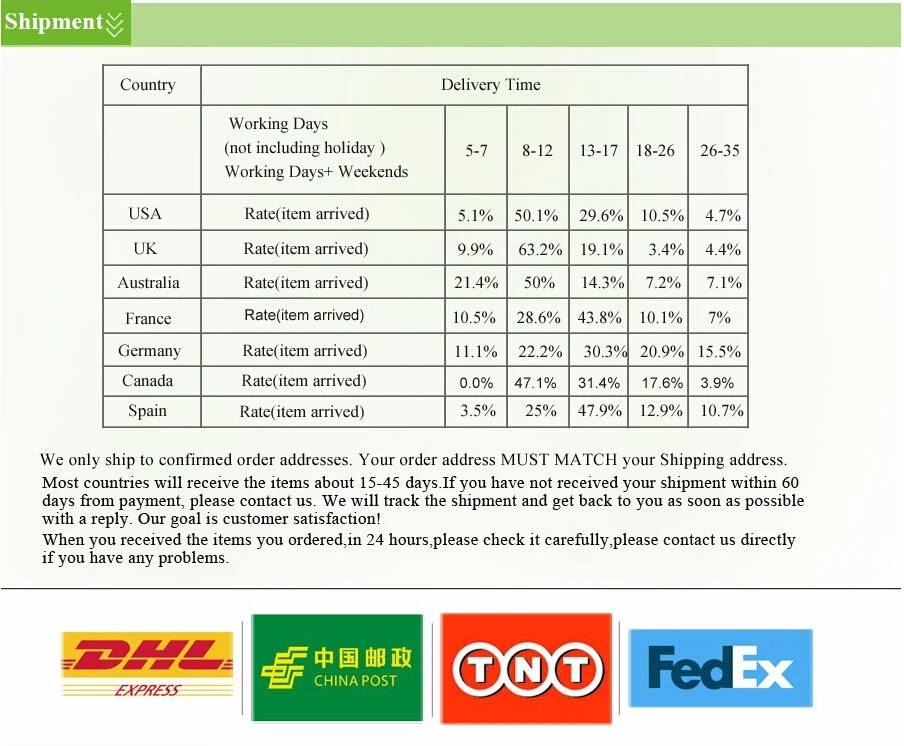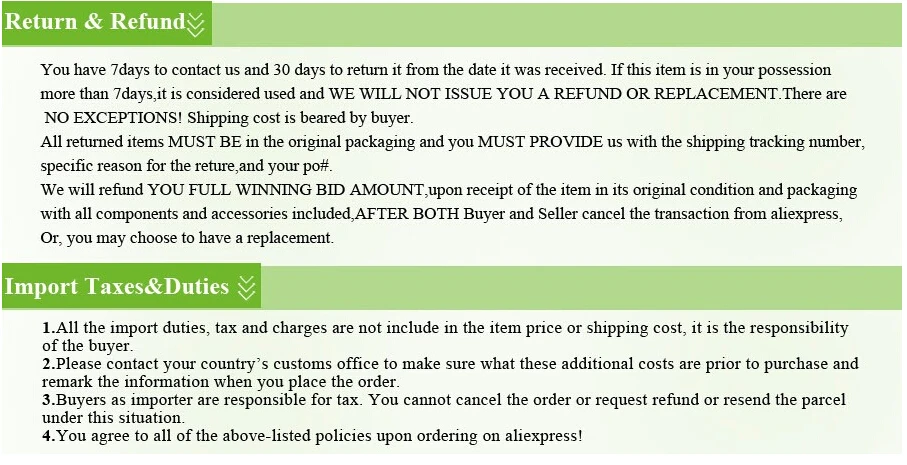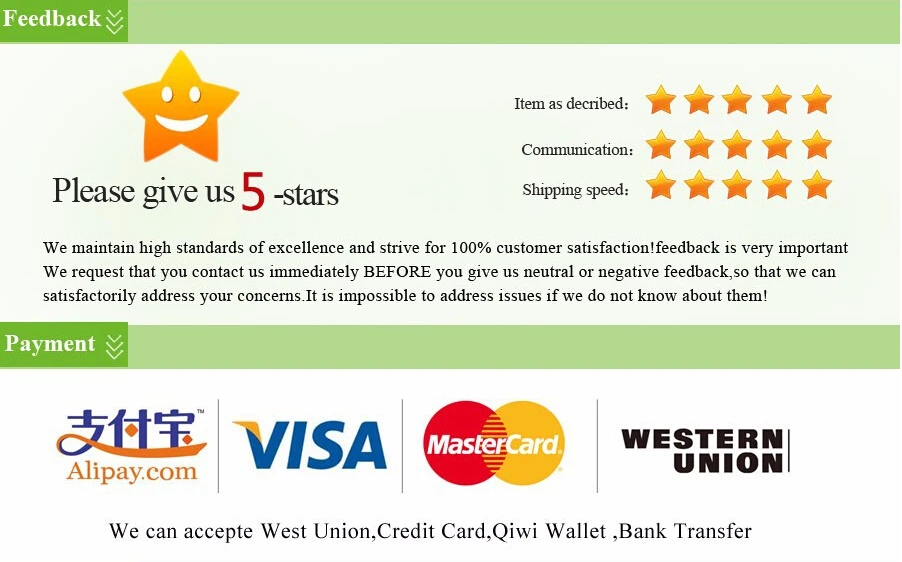# Customized mural wallpaper 3D sense of sight maple leaf pattern as background the porch corridor or enter hall (Other)

Regular price
\$150.94
Sale price
\$150.94
Regular price
Sold out
Unit price
per
Shipping calculated at checkout.

wallpaper price: \$ / square meter.    Not rolls.   1 square meter = 1pcs.   2 square meter=2pcs.   3 square meter =3pcs ...

Width x height = square meters = Order Quantity

Note: Please be careful to order 1㎡. Because a very small size

How to place the order?

If you measure in feet, you need to convert to a metric unit to place an order or give me information; I will give you calculate the correct order quantity.

Width x height = square meters (square feet)

1 square feet = 0.0929 square meters

For example: The sizes of your own wall :Width = 3.2m  Height = 2.8m

3.2m x 2.8m = 8.96 .The quantity You need to order is 9.If you need 7.1- 7.9 square meter, Please place the order of 8 square meters.

Tips :If we haven't got the detail wall size within 24 hours, we will produce by our default size as follow:

16 Default size :

Quantity 1pcs = 1 square meter = 140cm(W) x 70cm(H) (4'7" x 2'4") ft
Quantity 2pcs = 2 square meter = 200cm(W) x 100cm(H) (6'7" x 3'3") ft

Quantity 3pcs = 3 square meter = 220cm(W) x 140cm(H) (7'3" x 4'7") ft

Quantity 4pcs = 4 square meter = 250cm(W) x 160cm(H) (8'2" x 5'3") ft

Quantity 5pcs = 5 square meter = 280cm(W) x 180cm(H) (9'2" x 5'11") ft
Quantity 6pcs = 6 square meter = 300cm(W) x 200cm(H) (9'10" x 6'7") ft
Quantity 7pcs = 7 square meter = 330cm(W) x 210cm(H) (10'10" x 6'11") ft
Quantity 8pcs = 8 square meter = 360cm(W) x 230cm(H) (11'10" x 7'6") ft
Quantity 9pcs = 9 square meter = 380cm(W) x 240cm(H) (12'5" x 7'10") ft
Quantity 10pcs = 10 square meter = 400cm(W) x 250cm(H) (13'1" x 8'2") ft
Quantity 11pcs = 11 square meter = 420cm(W) x 260cm(H) (13'9'' x 8'6'') ft
Quantity 12pcs = 12 square meter = 440cm(W) x 270cm(H) (14'5" x 8'10") ft
Quantity 13pcs = 13 square meter = 460cm(W) x 280cm(H) (15'1'' x 9'2'') ft
Quantity 14pcs = 14 square meter = 480cm(W) x 290cm(H) (15'9'' x 9'6'') ft
Quantity 15pcs = 15 square meter = 500cm(W) x 300cm(H) (16'5'' x 9'10'') ft
Quantity 16pcs = 16 square meter = 500cm(W) x 320cm(H) (16'5" x 10'6") ft

Our price is based on size, different size, prices are different;Our prices are all included in transportation costs. Different national transportation companies are different and different in time.

If you buy 1-3 square meters, \$22 a square meter
Buy 4 to 10 square meters, is 16 dollars a square meter
Buy 11 to 30 square meters, 14 dollars a square meter
Buy 31 to 50 square meters, 12 dollars a square meter
Buy 51 to 100 square meters, 10 dollars a square meter
Buy more than 101 square meters, 8 dollars a square meter

If you customize other sizes, you can contact us to change the price for you.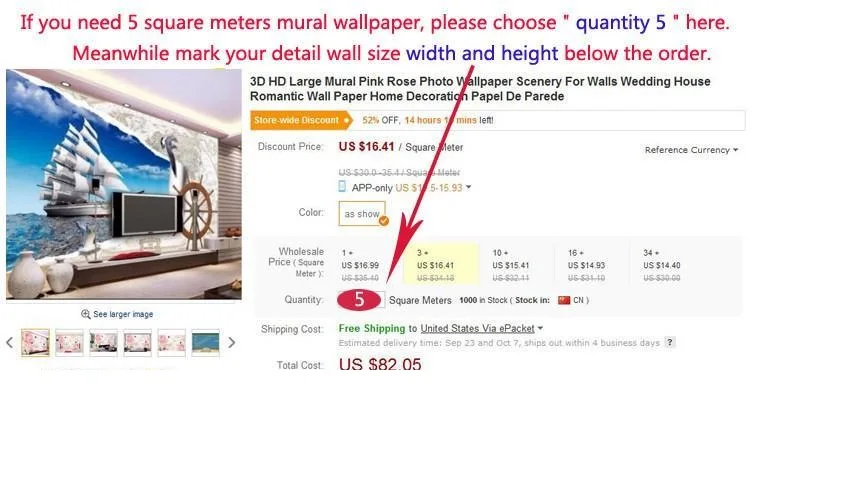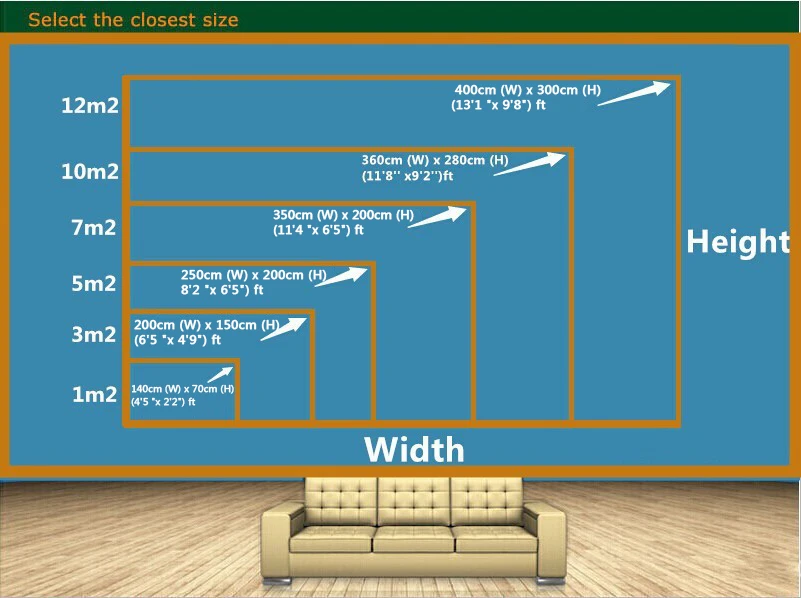Products display:

Shown effect with Computer-generated as below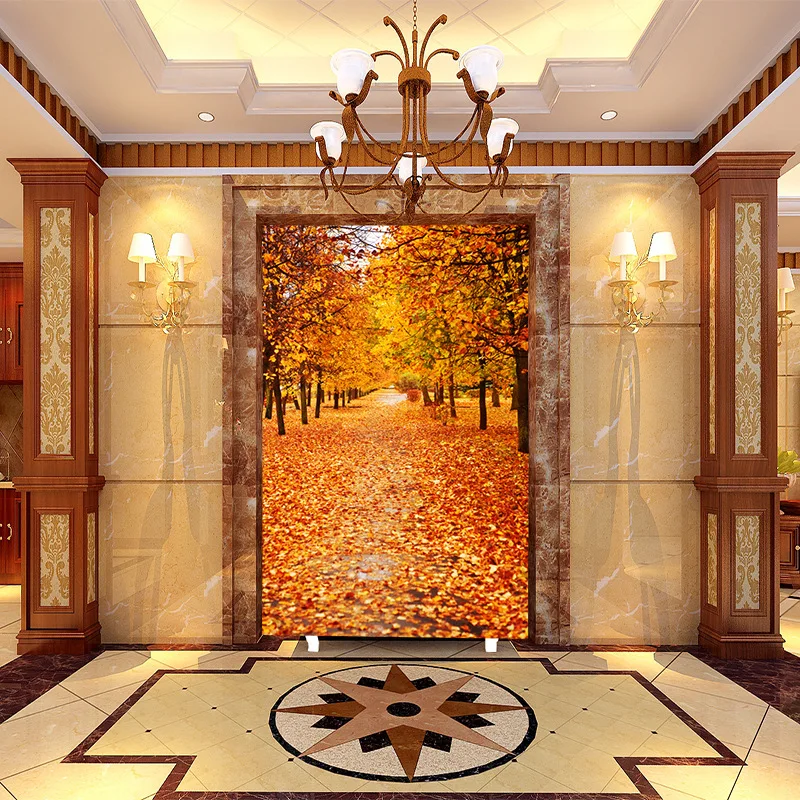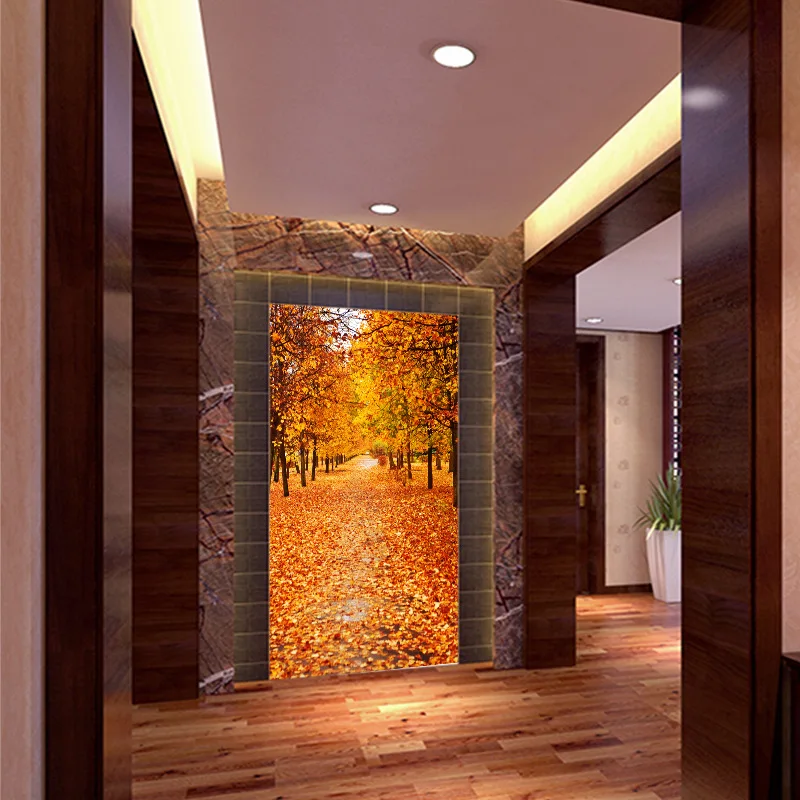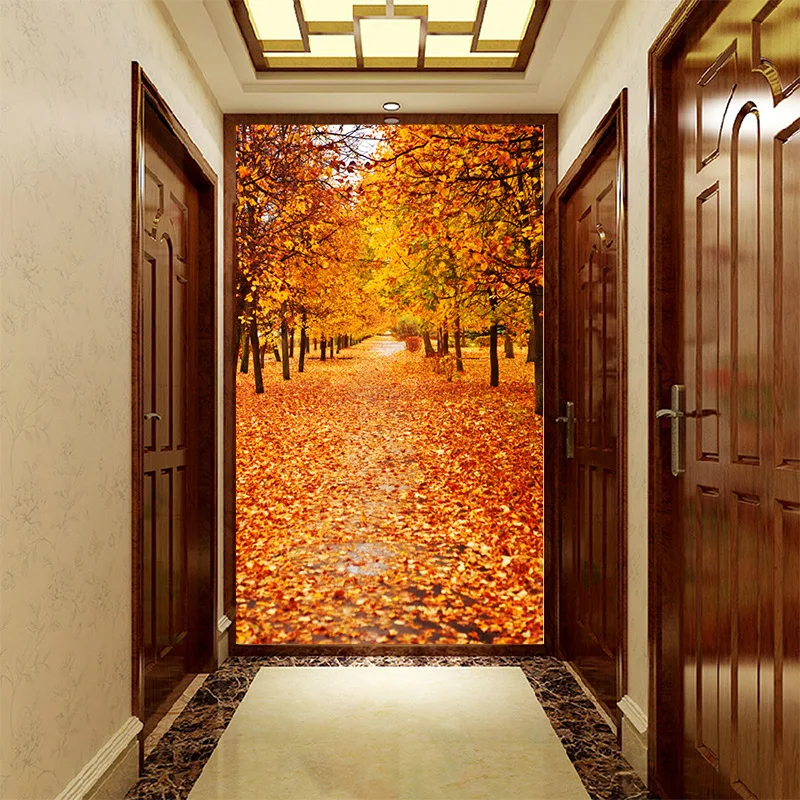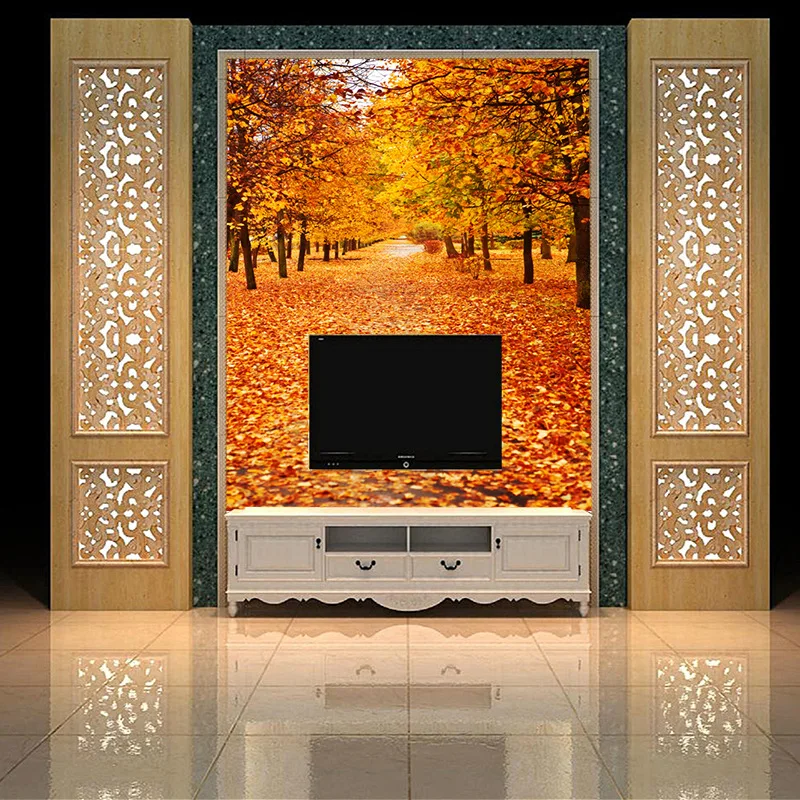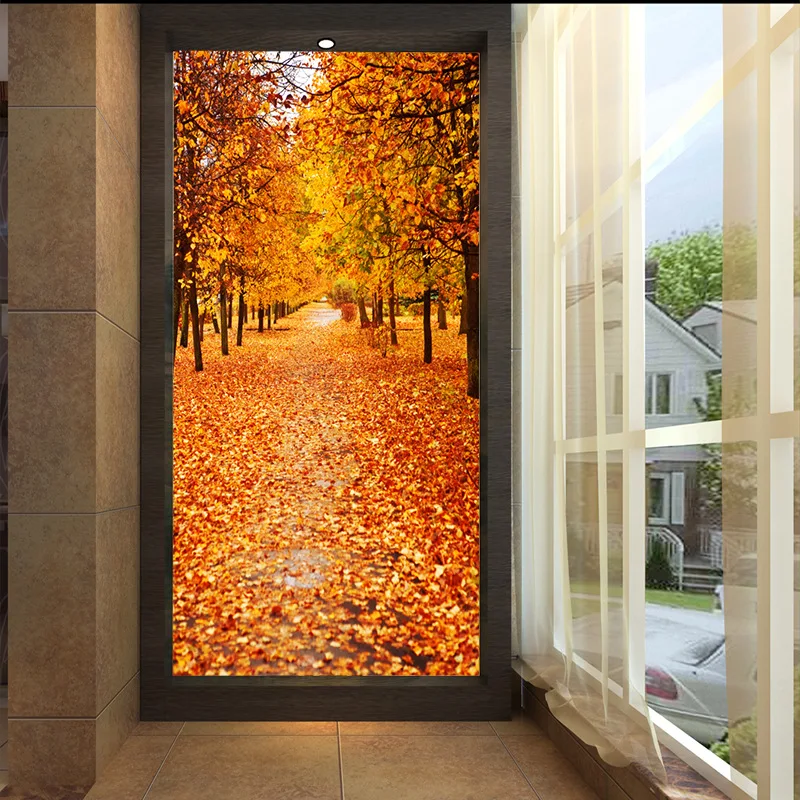Tips:due to a bit different dispaly by variety ,please be noticed.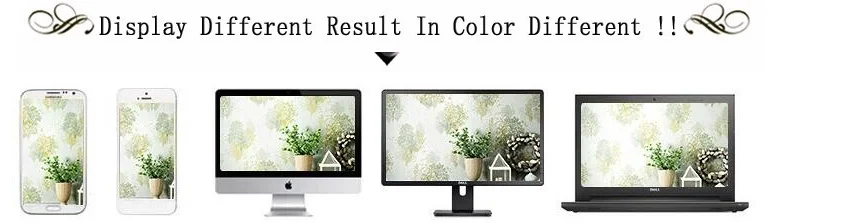Material: the highest-end material -5D three-dimensional texture silk cloth, clear, the best effect.